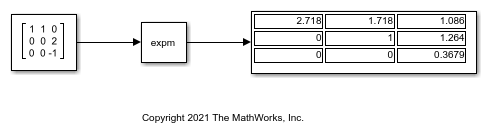# Compute Matrix Exponential

Compute the matrix exponential of an input matrix.

Open and run the model.The input matrix is a square matrix. The Matrix Exponential block computes the matrix exponential of the square matrix using a scaling and squaring algorithm with a Pade approximation. To view the implementation, look under the mask of the Matrix Exponential block by either clicking the down arrow on the block mask or by right-clicking the block and clicking Mask > Look Under Mask.